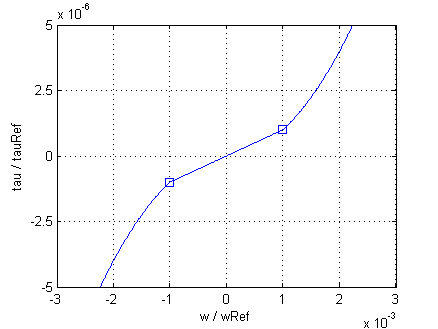#.Modelica.Electrical.Machines.Losses.Friction

## Information

The friction losses are considered by the equations

```   tau / tauRef = (+w / wRef) ^ power_w    for w > +wLinear
- tau / tauRef = (-w / wRef) ^ power_w    for w < -wLinear
```

with

```  tauRef * wRef = PRef
```

being the friction torque at the reference angular velocity `wRef`. The exponent `power_w` is approximately 1.5 for axial ventilation and approximately 2.0 for radial ventilation.

For stability reasons the friction torque `tau` is approximated by a linear curve

```  tau / tauLinear = w / wLinear
```

with

```  tauLinear = tauRef*(wLinear/wRef) ^ power_w
```

in the range `-wLinear ≤ w ≤ wLinear` with `wLinear = 0.001 * wRef`. The relationship of torque and angular velocity is depicted in Fig. 1Fig. 1: Friction loss torque versus angular velocity for `power_w = 2`

If it is desired to neglect friction losses, set `frictionParameters.PRef = 0` (this is the default).